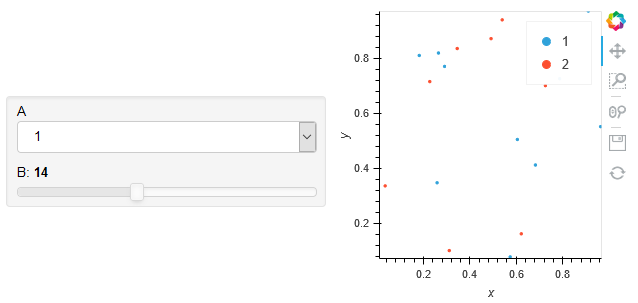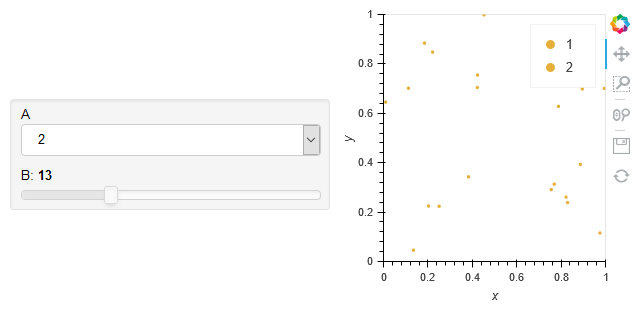# Strange behaviour when function returns NdOverlay to DynamicMap

I've encountered something very strange when having a function which generates an NdOverlay of Points to a DynamicMap, where the function is tied to panel widgets (I don't think the panel widgets are important).

The below code is a working example which produces the expected behavior. Whenever you change the widget values a new plot is generated with two sets of Points overlaid, with different colors and respective legend entries. Image shown below code.

``````a_widget = pn.widgets.Select(name='A', options=[1,2,3,4])
b_widget = pn.widgets.IntSlider(name='B', start=10, end=20, value=10)
widget_box = pn.WidgetBox(a_widget, b_widget, align='center')

@pn.depends(a=a_widget.param.value, b=b_widget.param.value)
def get_points(a, b):
return hv.NdOverlay({x: hv.Points(np.random.rand(10,10)) for x in range(1,3)})

points = hv.DynamicMap(get_points)

pn.Row(widget_box, points)
``````The second example shown below, is meant to demonstrate that in certain situations you might want to just simply return an empty plot and the way that I've done it in this example is done in the same way as in this example: http://holoviews.org/gallery/demos/bokeh/box_draw_roi_editor.html#bokeh-gallery-box-draw-roi-editor The result of this code is an empty plot as expected when `a == 1`, but when `a` has values other than `1`, the result is quite strange as illustrated in the image below the code.

1. The points all have the same color
2. When changing the slider for instance, some points are frozen and never changes, which is not the case in the above working example.
``````a_widget = pn.widgets.Select(name='A', options=[1,2,3,4])
b_widget = pn.widgets.IntSlider(name='B', start=10, end=20, value=10)
widget_box = pn.WidgetBox(a_widget, b_widget, align='center')

@pn.depends(a=a_widget.param.value, b=b_widget.param.value)
def get_points(a, b):
if a == 1:
return hv.NdOverlay({None: hv.Points([])})
else:
return hv.NdOverlay({x: hv.Points(np.random.rand(10,10)) for x in range(1,3)})

points = hv.DynamicMap(get_points)

pn.Row(widget_box, points)
``````• Hi Martin, it's probably best to post a link to your SO-question here: gitter.im/pyviz/pyviz so the devs of holoviews can help you with this (and other questions) – Sander van den Oord Nov 29 at 10:30
• Hi. Thanks for the tip. I've joined the room and asked for help :) – Martin Rindarøy Nov 29 at 16:01

While I can not help the observed issue with `NdOverlay`, creating plots with or without content can be done with the help of `Overlay`.

As `b_widget` is never used in your code, I removed it for simplicity.

``````a_widget = pn.widgets.Select(name='A', options=[1,2,3,4])
widget_box = pn.WidgetBox(a_widget, align='center')

@pn.depends(a=a_widget.param.value)
def get_points(a):
images = []
if a == 3:
images.append(hv.Points(np.random.rand(10,10), label='None'))
else:
for x in range(1,3):
images.append(hv.Points(np.random.rand(10,10), label=str(x)))
return hv.Overlay(images)

points = hv.DynamicMap(get_points)

pn.Row(widget_box, points)
``````

The way how to use NdOverlay that is described in the documentation for NdOverlay is different to your approach, this might be a reason for the observed problems.

Anyway, to narrow down which part of the code is responsible for the observed issue, I removed all code that is not necessary to reproduce it.

For clarity, I renamed the values of `a`, and I also made sure, that a start value for `a` is provided.

It turned out while testing the code, that the `if`-`else`-statement is neither important, so I removed that too.

And just to make sure, that variables behave like expected, I added some `print`-statements.

This gives the following minimal reproducable example:

``````a_widget = pn.widgets.Select(name='A', value='Test', options=['Test','Test1', 'Test2'])

@pn.depends(a=a_widget.param.value)
def get_points(a):
dict_ = {}
dict_[str(a)] = hv.Points(np.random.rand(10,10))

print(dict_)
overlay = hv.NdOverlay(dict_)
print(overlay)

return overlay

points = hv.DynamicMap(get_points)

# using the server approach here to see the outpout of the
# print-statements
app = pn.Row(a_widget, points)
app.app()
``````

When running this code, and choosing the different options in the select widget, it turns out that option `Test` is not updated, once one of the options `Test1` and `Test3` have been choosen.

When we change the default value in the first line like this

``````a_widget = pn.widgets.Select(name='A', value='Test2', options=['Test','Test1', 'Test2'])
``````

now `Test2` is not updated correctly.

So it looks like this is an issue of `DynamicMap` using `NdOverlay`. So I suggest you report this issue to the developers (if not already done), either wait for new release or use a different approach (e.g. as shown above).

• `b_widget` is used...(?) Even so, my example strongly resembles the example on holoviews.org which I'm linking to in the question. – Martin Rindarøy Dec 6 at 3:28
• @ Martin Rindarøy: I've updated my answer. It contains now a part based on your code and the linked example. – mdk 2 days ago